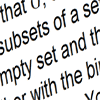#### You may also like### Small Groups

Learn about the rules for a group and the different groups of 4 elements by doing some simple puzzles.### An Introduction to Galois Theory

This article only skims the surface of Galois theory and should probably be accessible to a 17 or 18 year old school student with a strong interest in mathematics.### Groups of Sets

The binary operation * for combining sets is defined as the union of two sets minus their intersection. Prove the set of all subsets of a set S together with the binary operation * forms a group.

# What's a Group?

##### Age 16 to 18Challenge Level

This problem is about infinite groups. We first meet groups when we do simple number work and they are useful in much more general applications. To use groups in higher mathematics we need a precise definition.

A group is a set of elements together with a binary operation and four defining properies (see the notes). Some groups are commutative (Abelian) and other groups are not.

(a) Convince yourself that the set of integers with the operation of addition satisfies the four given conditions and so forms a group. Why does the set of natural numbers (positive integers) with the operation of subtraction not form a group?

(b) Convince yourself that the set of positive rational numbers with the operation of multiplication forms a group. Why does the set of positive rational numbers with the operation of division not form a group?

(c) Why does the set of positive integers with the operation of multiplication not form a group?

(d) Why does the set of positive even integers with the operation of multiplication not form a group?

(e) The set of integers with the operation * defined such that $m*n = m + n + 1$ is a group. Find the identity element and the inverse of the element $m$.

(f) The set of integers with the operation * defined by $m*n = m + (-1)^m n$ is a group. Find the identity element and the inverse of the element $m$.

(g) The set of all real numbers excluding only the number -1 together with the operation $x*y = xy + x + y$ is a group. Find the identity and the inverse of the element $x$.